In which of the following crystals alternate tetrahedral voids are occupied

1. NaCl

2. ZnS

3. CaF2

4. Na2O

Concept Questions :-

High Yielding Test Series + Question Bank - NEET 2020

Difficulty Level:

In a metal oxide, the oxide ions are arranged in hexagonal close packing and metal ions occupy two third of the octahedral voids. The formula of the oxide is

1. MO

2. M2O3

3. MO2

4. M2O

Concept Questions :-

Density/Formula/packing fraction/ Semiconductors
High Yielding Test Series + Question Bank - NEET 2020

Difficulty Level:

A crystal is made of particle X, Y & Z. X forms FCC packing, Y occupies all octahedral voids of X and Zoccupies all tetrahedral voids of X, if all the pariticles along one body diagonal are removed then the formula of the crystal would be –

(1) XYZ2

(2) X2YZ2

(3) X5Y4Z5

(4) X5Y4Z8

Concept Questions :-

Density/Formula/packing fraction/ Semiconductors
High Yielding Test Series + Question Bank - NEET 2020

Difficulty Level:

In a hypothetical solid C atoms are found to form cubical close packed lattice. Aatoms occupy all tetrahedral vioids& B atoms occupy all octahedral voids. A and B atoms are of appropriate size, so that there is no distortion in CCP lattice of C atoms. Now if a plane as shown in the following figure is cut.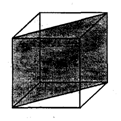Then the cross section of this plane will look like.

(1)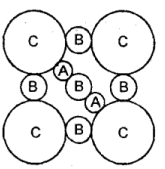(2)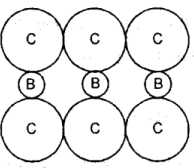(3)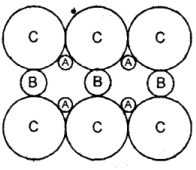(4)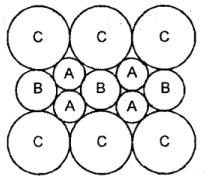Concept Questions :-

High Yielding Test Series + Question Bank - NEET 2020

Difficulty Level:

In hexagonal close packing of sphere in three dimensions.

(1) In one unit cell there are 12 octahedral voids and all are completely inside the unit cell.

(2) In one unit cell there are six octahedral voids and all are completely inside the unit cell.

(3) In one unit cell there are six octahedral void and of which three are completely inside the unit cell and other three are partially inside the unit cell.

(4) In one unit cell there are 12 tetrahedral voids, all are completely inside the unit cell.

Concept Questions :-

Density/Formula/packing fraction/ Semiconductors
High Yielding Test Series + Question Bank - NEET 2020

Difficulty Level:

Diamond has face-centred cubic lattice. There are two atoms at (0,0,0) and $\left(\frac{1}{4},\frac{1}{4},\frac{1}{4}\right)$coordinates.The ratioof the carbon-carbon bond distance to the edge of the unit cell is

(1) $\sqrt{\frac{3}{16}}$

(2) 4

(3) $\frac{1}{4}$

(4) $\frac{1}{\sqrt{2}}$

Concept Questions :-

Density/Formula/packing fraction/ Semiconductors
High Yielding Test Series + Question Bank - NEET 2020

Difficulty Level:

Spinel is an important class of oxides consisting of two types of metal ions with the oxide ions arranged inCCP pattern. The normal spinel has 1/ 8 of the tetrahedral holes occupied by one type of metal ion and 1/2 of the octahedral holes occupied by another type of metal ion. Such a spinel is formed by Zn2+, A13* and 02-with Zn2+ in the tetrahedral holes. If CCP arrangement of oxide ions remains undistorted in the presence of all the cations, formula of spinel and fraction of the packing fraction of crystal are respectively :

(1) ZnAl2O4 , 77%

(2) ZnAl2O4, 74%

(3) Zn2AlO4, 74%

(4) Zn3Al2O6,74%

Concept Questions :-

Introduction and Type of Crystal System
High Yielding Test Series + Question Bank - NEET 2020

Difficulty Level:

What is the maximum number of layers of atoms in close packed planes that will lie within two imaginaryparallel planes having a distance between them of 13$\sqrt{\frac{2}{3}}$ R (where R is the radius of atom) in the coppercrystal (FCC) ? Consider the atoms to be within the parallel planes if their centresare on or within the two parallel planes.

(1) 5

(2) 6

(3) 7

(4) 8

Concept Questions :-

Introduction and Type of Crystal System
High Yielding Test Series + Question Bank - NEET 2020

Difficulty Level:

A transition Metal Mcan exist in two oxidation states +2 and +3.It forms an oxide whose experimentalformula is given by MxO where x < 1. Then the ratio of metal ions in +3 state to those in +2 state in oxide is given by :

(1) $\frac{1-x}{1+x}$

(2) 1 + 2X

(3) 1 + $\frac{x}{2}$

(4) $\frac{2\left(x-1\right)}{3x-2}$

High Yielding Test Series + Question Bank - NEET 2020

Difficulty Level:

Analysis show that nickel oxide consist of nickel ion with 96% ions having d8 configuration and 4% having d7 configuration. Which amongst the following best represents the formula of the oxide.

(1) Ni1.02O1.00

(2) Ni0.96O1.00

(3) Ni0.96O0.98

(4) Ni0.98O1.00